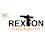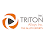### Understanding Geometric Nonlinearity - 1 by Kotur Raghavan

Preamble

Any structure deforms when subjected to loads. The basic question is whether the deformations affect the structural behavior to any practically significant extent. If they do, we know that the small deformation theories are inadequate and it is necessary to invoke large deformation formulation to obtain acceptable results. In other words, one has to invoke the  “Geometric Nonlinearity” option while using FEA packages to obtain good results. The general thumb-rule is that when the deformations are comparable to the dimensions of the structure, the effects of geometric nonlinearity become noticeable.

In the small deformation theory or linear elasticity, the basic assumption is that the displacements in orthogonal directions are uncoupled. In Cartesian geometry, the displacement fields X- and Y- directions are independent of one another. In the cylindrical coordinate system, the displacement fields in the radial and tangential directions are assumed to be independent of one another. In the present article, a simple example of a simply supported bar is chosen to demonstrate the effect of the coupling of displacement fields.

Case Study Problem and Modeling Options

The problem for the study is shown in the figure below.

Fig. 1

Only the left half of the beam is considered for analysis. Two-dimensional plane stress elements are chosen. At the plane of symmetry, the axial displacements are constrained. At the supported end, two different sets of constraints are applied. They are described in the figure below.

Fig. 2

The constraint sets are referred to as C1 and C2. The black dots denote the nodes where the transverse displacements are constrained. For both the models, linear as well as nonlinear analyses have been carried out. The four models will be referred to as LC1, LC2, NLC1, and NLC2.

Results of Analysis

The computed values of the maximum deflections for the four cases are summarized in the table below.

Table 1

The plots of deflections against the fraction of the applied load are given in the figure below.

There is no difference between the responses for the two linear cases. For the NLC1 case, we observe a small amount of nonlinearity effect. The nonlinearity effect is noticeable when the deflection is comparable to the beam thickness. For the NLC2 case, however, the effect of geometric nonlinearity is more pronounced. This is due to the effect of coupling of displacements in orthogonal directions which is inherent in formulations applicable to large deformations. This aspect is discussed in the next section.

Analysis

In the figure below, the line AB corresponds to the supported edge before deformation. Due to the applied load and the resultant deformation, it will take a new position that corresponds to the broken line A1-B1.

Fig. 5

Due to requirements of geometric compatibility, points A and B will have non-zero displacements in the vertical (-) direction. This requirement does not arise in a linear analysis. In the case of nonlinear analysis, the constraint set C1 permits the points A and B to take the new positions A1 and B1.

However, for the nonlinear analysis involving the constraints C2, points A and B do not have the freedom to occupy the positions as indicated. Instead, points A and B are restrained to remain along lines PP and QQ respectively. This factor adds additional stiffness. This additional stiffness is dependent on the extent of deformation and hence can be considered to be the “geometric nonlinear” effect. From the figure, it can be noticed that this effect starts manifesting at a deflection-to-thickness ratio of 0.5.

------------------------------------------------------------------------------------------------------------------------

1.You have created a great blog . we are Manufacturer of Plates & Sheets

2.The format and details are described in great detail. I appreciate everything you've done. Thank you very much. please go to Specialized Steel Pipes

3.There is a lot of detail described. We are a Supplier of UNS S32750 Round Bar

4.This is a very informative piece. I appreciate you sharing this helpful data. Please keep this kind of reporting coming.  Our UNS N08904 Seamless Pipe is the best in the industry. To find out more, check out.